# Circle

## Introduction

In this article we are going to study the geometric figure circle, its characteristics and mathematical equation.

## Definition of a circle

A circle is a geometric figure in which all its points are at the same distance from a given point called the center, this distance is known as the radius of the circle.

## Equation of a Circle

Knowing the mathematical expression of a circumference we will be able to draw it in the Cartesian plane and later use it in our projects of programming and development of videogames.

### Cartesian coordinates

The expression that defines a circle in Cartesian coordinates is the following:

(1)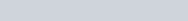Where R is the radius of the circumference and its center is located at point (a,b) of the Cartesian plane.

In this case to draw the circle we must know the range of values of the variables X and Y. For example, let’s consider the circle unit centered in (0,0):

(2)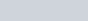If we draw a circle of radius 1 with center in (0,0) we can see that both the values of X and Y will be in the interval [-1,1]. By choosing a value from that interval and assigning it to one of the variables we will be able to clear the equation and obtain the value of the other variable.

### Parametric coordinates

The expression of a circumference in the parametric coordinate system may also be useful, since the range of variation of the parameter is infinite since it is defined with periodic functions. The expression of a circle is given by the following system of equations.

x=a+r.cos(t)

y=b+r.sin(t)

(3)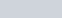By varying the t parameter between 0 and 2π we obtain all the points of the circumference.

As we mentioned before the parameter t can take values from less infinite to more infinite and will always return some point of the circumference, because the sinuses and cosines are periodic functions.

## Circle and mathematical functions

It must be borne in mind that there is no mathematical function that defines the circle since, by definition, a function is an expression in which it is fulfilled that, for each value of the independent variable, there is only one value for the dependent variable.

In others for it to be a function we must be able to draw a vertical line anywhere on the graph and this should cut the function into a single point. This does not happen in the circle, since if we take for example the line coincident with the Y axis, we see that it cuts the circle unit centered in (0,0) in two points.

What we can do is clear the variable and in equation 1.

(4)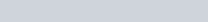The absolute value arises when taking the square root of an expression that is elevated to the square, this gives rise to two possible values for that expression, one positive and the other negative, that define the lower and upper cap of the circumference.

### Semicircle – Concave from below

The expression of the function that represents the top semicircle of a generic circle is:

(5)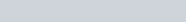### Semicircle – Concave from above

The expression of the function that represents the bottom semicircle of a generic circle is:

(6)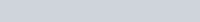### Perimeter of a circle

The perimeter of a circle is the length of its boundary, let’s imagine that we make a mark in a point of the circle, we place that point in the 0 of a line and we make it turn forward, the point where the mark touches the line again is the value of the perimeter of the circumference. Mathematically it can be calculated as:

(7)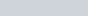Pi has an approximate value of 3.1415 and r is the radius of the circumference.

### Area of a circle

The area of a circle is the result of multiplying the number Pi by the radius of the circle squared. Mathematically:

(8)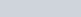Scroll to Top
Secured By miniOrange